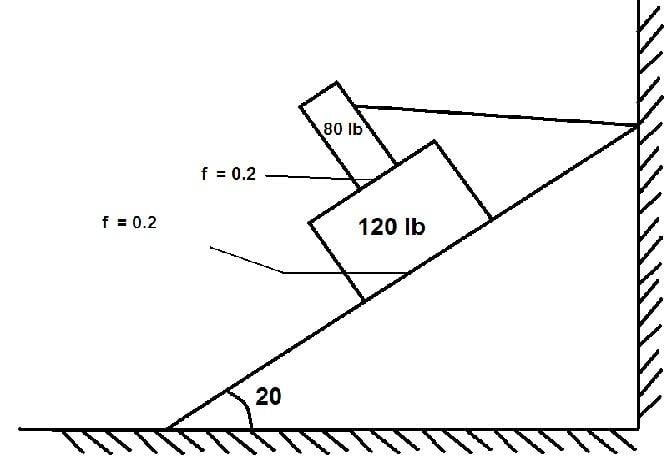# Friction of Two Blocks and Rope Problem

FairyChatMan

## Homework Statement

Two blocks are positioned according to the figure. Block A (80 lb) is attached to a rope as such that it doesn't slide or fall from block B (120lb). Compute the smallest value of Force to be applied on Block B for it to not move parallel to the inclined plane.## Homework Equations

F = fN
N = Wcos$$\theta$$

## The Attempt at a Solution

Here's how I did, I assumed that Block B is sliding downwards parallel to the inclined plane.

Then I compute the Frictional force between block A and B, so

W = 80 lb
N = 80cos20
Friction forceA&B = 0.2(80cos20) direction is upwards parallel to inclined plane, because it will resist the downward sliding motion of Block B.

Then compute for Friction force between Block B and plane, so

W = 80 +120 (is this correct?)
N = 200cos20
Friction forceB&plane = 0.2(200cos20) direction same with previous Ff.

Then I compute for the sliding magnitude of Block B, assuming it will slide because of none external force acting on it other than friction

F = 120sin20 (force not applying frictions)
F2 = 120sin20 - (0.2(80cos20) + 0.2(200cos20)) = Answer

I equate F2 as my answer because that's the only force left in the system needed to be countered..... so my force needs to be acting upward...

When I substituted all values.... it gives me a negative answer force... so does that mean... I'm wrong?